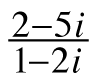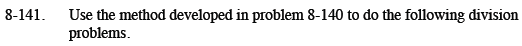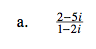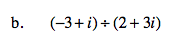Home > CCA2 > Chapter 8 > Lesson 8.3.2 > Problem8-141

8-141.
1. Use the method developed in problem 8-140 to do the following division problems. Homework Help ✎

1.2. (−3 + i) ÷ (2 + 3i)Multiply by a Giant One using the complex conjugate of the denominator.

Write the result as a complex number, a + bi.

$\frac{12}{5} - \frac{1}{5}i$Rewrite the division problem in fraction form.
See part (a) for more help.